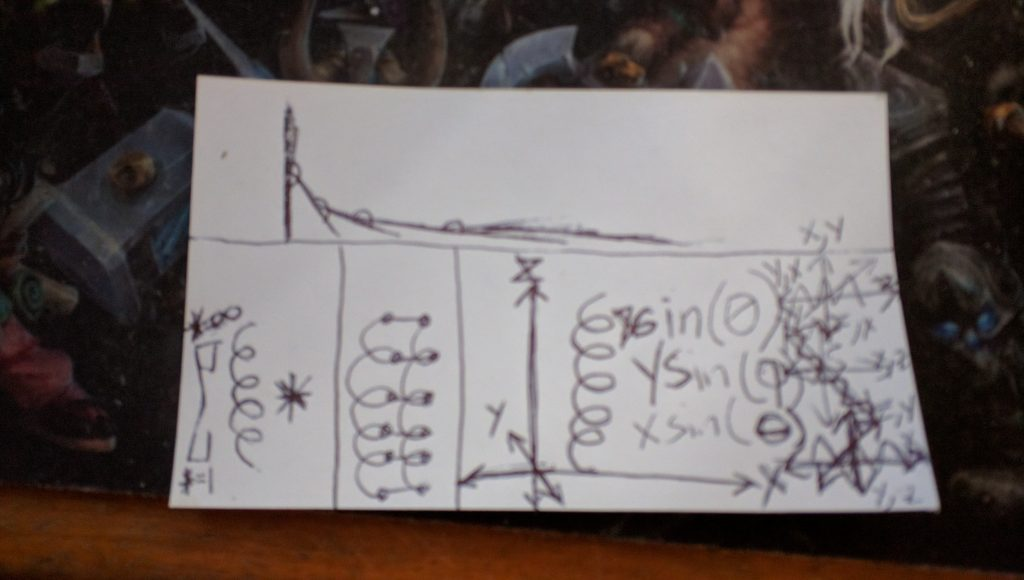## Double Helix is the Cure for KinkyPolySpringPendulOSussAtIonAtOr

Infinite legs, infinite toes, infinite arms, infinite fingers, infinite heads, infinite zibs, infinite sphincters, infinite sound sensors, infinite vision sensors, infinite temperature sensors, infinite tensile strength sensors, infinite center of gravity sensors, infinite friction sensors, infinite sense and force sensors in general, infinite actuators, infinite grace.

Super Spheres.

Infinitely expanding linear dot matrix.

*

LinearlySinusoidalSinusoids and LinearlyPositionallyFlexive shapes such as y=x^2 are intermediaries of deconstruction and creation as time passes so as to change the shapes of half-finished, imperfect scaffolds into *.

Minimally Reduplicated Half Shape of Line: •}]=[{•

Treefingers

Minimally Reduplicated Full Shape of Line with Perspective Shift: [{•}]=:}]

Cactal fractals.

Double helix is the cure for kinky.

5 minute drawing:Suggested Listening: Tool – Lateralus

Next: -1 * -1 = +1 »
« Previous: x% off

This site uses Akismet to reduce spam. Learn how your comment data is processed.